×

Search anything:

# Calculator console application in Python

#### PythonGet this book -> Problems on Array: For Interviews and Competitive Programming

We build a console calculator application using Python Programming Language in this article.

1. This application can perform basic arithmetic operations like addition, subtraction, multiplication and division.
2. It can evaluate simple mathematical expressions like 8 + 9 - 6 ** 7 (** stands for power operation)
3. The application can store the resultant value after a calculation. The user can use this value in further calculations by using the letter 'p' in its place.
if the last calculated value was 5, then 'p' can be used to refer to this value
then p + 5 would equal 11.

## Contents

1. Taking input from user
2. Scanning input from user
4. Subtraction
5. Mutliplication
6. Division
7. Evaluating expressions

## Taking input from the user

• Print the instructions: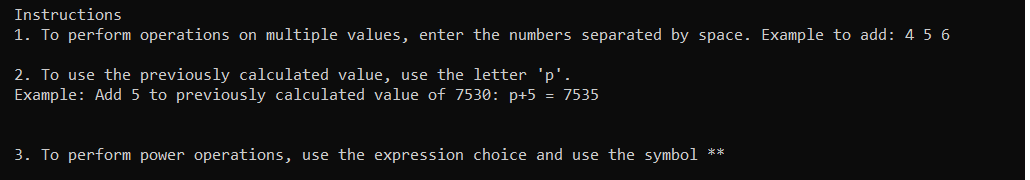• Print the choices and take the input choice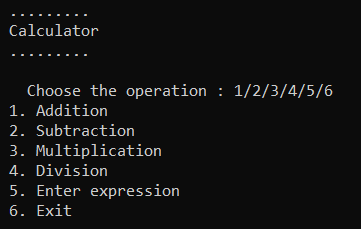• To add a set of numbers, user has to choose option 1 and to find out the difference of numbers, user should select option 2.

• Option 3 multiplies numbers and option 4 is used to perform division.

• To input an expression to be evaluated, the user has to choose option 5 and to exit, user has to choose option 6.

• The calculator application prompts the user to enter the operation and operands or the expression till the exit option 6 is entered.

• The user can use the previously computed value in the following calculations. Use a variable to hold the value of the calculation so that it can be used in the next calculation. Initially variable will hold the value '0'.
`prev_value = 0`

``````while(True):
print("\n ......... \n Calculator\n .........\n")

try:
operation = int(input("   Choose the operation : 1/2/3/4/5/6 \n 1. Addition \n 2. Subtraction \n 3. Multiplication \n 4. Division \n 5. Enter expression \n 6. Exit \n "))
if operation not in {1,2,3,4,5,6}:
print("!!! Enter only numbers 1/2/3/4/5/6 !!!")
continue
except ValueError:
print("!!! Enter only numbers 1/2/3/4/5/6 !!!")
continue
``````
• If any input other than a number is entered, it will raise an exception and prompt the user to enter only numbers.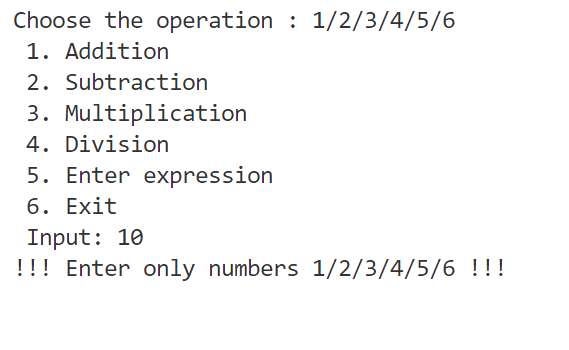## Scanning input from user

1. After the user chooses among operations 1,2,3,4,5, we need to take in the operands values to perform the calculations.
2. input("Enter values: ") takes the input values from the user in the form of a string. Since we're using space as the separator between multiple values, we split the input into different values by using split() method.
3. split() method splits a string into a list based on a separator. By default it's any whitespace.
4. So input("Enter values: ").split() returns a list
Example:
```Addition -> input("Enter values: ")      44 7 9
input("Enter values: ").split()       List - ['44', '7', '9']```
5. The user can input the letter 'p' to use the previously calculated value. So we traverse through the list and replace 'p' with the previous value.
6. Now we need to convert the string values in the list to integer values so that operations can be performed on them.
``````
#since division and expression take input differently
if operation not in {4, 5,6}:
try:
x = [x for x in input("Enter values: ").split()]

#replacing 'p' with prev_value
for index in range(len(x)):
if x[index] == 'p':
x[index] = prev_value

#creating list of integers
num_list = [int(x) for x in x]

except ValueError:
print("Enter a number for valid input!\n")
continue
``````

• The user can input as many operands or numbers to be added as required.
• The numbers entered should be separated by a space, like 6 14 98
• Traverse through the integer input list and perform addition. Store the calculated value in prev_value variable.
``````if operation == 1:
sum=0
for item in num_list:
sum += item
print("Sum: ", sum)
prev_value = sum
``````

Output: 2589 + 325832 = 328421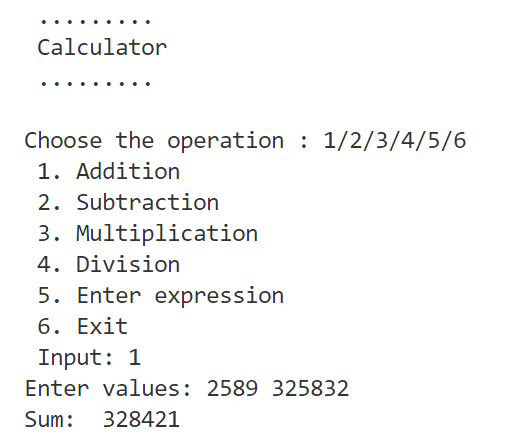## Difference

• The user can input as many operands or numbers to be added as required.
• The numbers entered should be separated by a space, like 6 14 98
If expression is 8-9 then it should be input as 8 -9
• Store the calculated value in prev_value variable.
``````elif operation == 2:
diff=0

for item in num_list:
diff += item
print("Difference: ", diff)
prev_value = diff
``````

Output: 8-9-7+8-17 = -17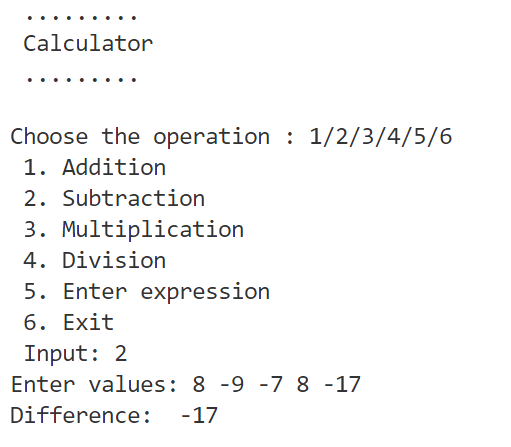## Multiplication

• The user can input as many operands or numbers to be added as required.
• The numbers entered should be separated by a space, like 7 9 0
• Traverse through the integer input list and perform multiplication. Store the calculated value in prev_value variable.
`````` elif operation == 3:
product=1

for item in num_list:
product *= item
print("Product: ", product)
prev_value = product
``````

Output: 8903 * 209409 = 1864368327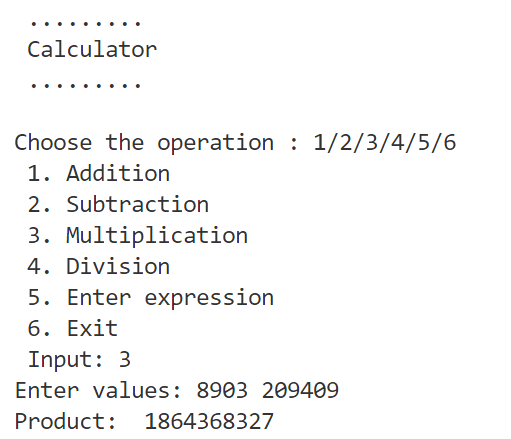## Division

• The user needs to enter the dividend and divisor. If either of the numbers is input as 'p', then we replace with the previously calculated value.
• The division result is rounded off to 5 places.
`````` elif operation == 4:
num1 = input("Enter dividend ")
num2 = input("Enter divisor: ")

if num1 == 'p':
num1 = prev_value

elif num2 == 'p':
num2 = prev_value

div = int(num1)/int(num2)

#rounded result
round_div = round(div, 5)

print("Result: ", num1, "/", num2, "=", round_div)
prev_value = round_div
``````

Output: 1864368327 / 29305 = 63619.46176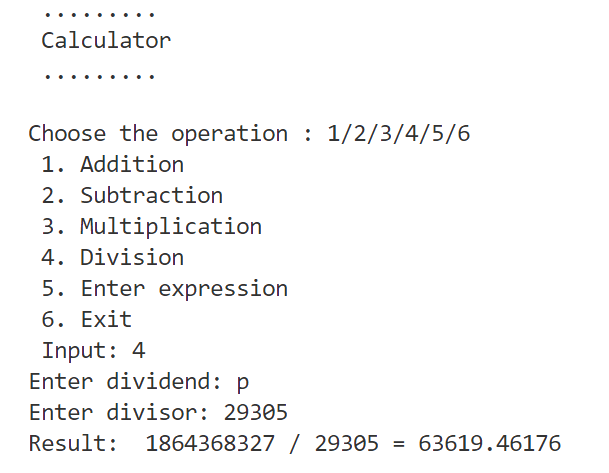## Expression

• The user can input any expression, like 7 ** 9 + 6 (7^9+6)

• eval() function is a built-in function that can evaluate mathematical expressions.
eval(expression [, globals[, locals]])
expression argument is a string. It is parsed then evaluated as a Python expression and the result is returned. globals and locals are optional arguments. globals argument is a dictionary that gives eval() a global namespace. locals argument also is a dictionary and it holds variables of local names that eval() evaluates.

• Take input from the user and transform into a list. Check if any item in the list equals 'p', if so, replace with the variable prev_value.

• Convert the list back into a string using the join() method.
The join() method joins all items of an iterable object into a single string.
A separator string should be specified, like exp = "".join(exp), "" is the separator.

• This string can now be passed as an argument to the eval() function and the result can be printed.

``````elif operation == 5:
try:
exp = input("Enter the expression \n")

exp = list(exp)

for letter in range(len(exp)):
if exp[letter] == 'p':
exp[letter] = str(prev_value)
exp = "".join(exp)

result = eval(exp)
print("Result: "+ exp + ' = ' + str(result))
prev_value = result

except SyntaxError:
print("Enter a valid expression!\n")
continue
``````

Output: 2932024 - p + 28 ** 9 = 2932024-63619.46176 (p) +28 ** 9 = 10578458821812.54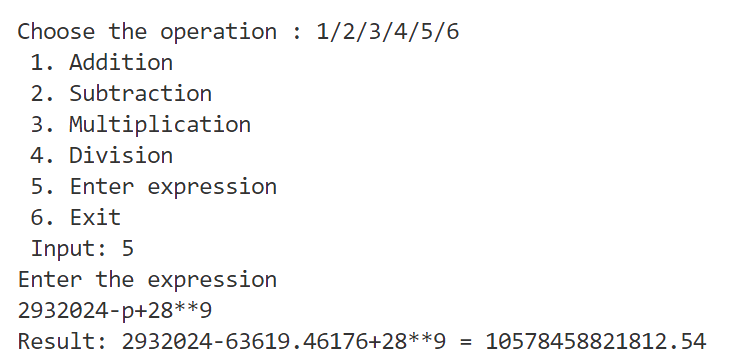• The user can exit the calculator by choosing the operation input to be 6 and the program calls the exit() function and thus ends.

With this article at OpenGenus, you can develop a basic console calculator using Python.#### Sai Siri Chandana Namala

Graduate student of Computer Science at JNTUH College Of Engineering